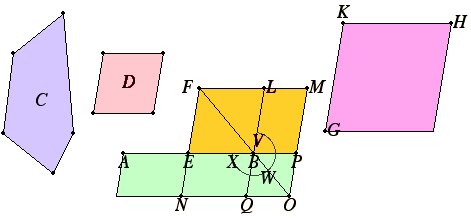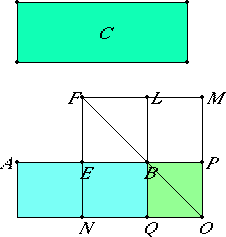# Proposition 29

To apply a parallelogram equal to a given rectilinear figure to a given straight line but exceeding it by a parallelogram similar to a given one.

Let C be the given rectilinear figure, AB be the given straight line, and D the parallelogram to which the excess is required to be similar.

It is required to apply a parallelogram equal to the the rectilinear figure C to the straight line AB but exceeding it by a parallelogram similar to D.VI.25

Bisect AB at E. Describe the parallelogram BF on EB similar and similarly situated to D, and construct GH equal to the sum of BF and C and similar and similarly situated to D.

Let KH correspond to FL and KG to FE.

Now, since GH is greater than FB, therefore KH is also greater than FL, and KG greater thanFE.

Produce FL and FE. Make FLM equal to KH, and FEN equal to KG. Complete MN. Then MN is both equal and similar to GH.

But GH is similar to EL, therefore MN is also similar to EL, therefore EL is about the same diameter with MN.

Draw their diameter FO, and describe the figure.

Since GH equals the sum of EL and C, while GH equals MN, therefore MN also equals the sum of EL and C.

Subtract EL from each. Therefore the remainder, the gnomon XWV, equals C.

Now, since AE equals EB, therefore AN equals NB, that is, LP.

Add EO to each. Therefore the whole AO equals the gnomon VWX.

But the gnomon VWX equals C, therefore AO also equals C.

VI.24

Therefore the parallelogram AO equal to the given rectilinear figure C has been applied to the given straight line AB but exceeding it by a parallelogram QP similar to D, since PQ is also similar to EL.

Q.E.F.

## Guide

The construction in this proposition is a generalization of that described in the Guide for II.6. In that proposition, the figure D is a square.

#### The outline of a simplified proof for rectangles

Like the last proposition, this one is more easily understood when the given parallelogram D is a square.In that case of this proposition a rectangle AO equal to a given rectilinear figure C is applied to a given straight line AB but exceeds it by a square (BQOP in the figure). So the rectangle being laid alongside the line extends past the end of the line AB, but the part that extends beyond the end is a square. For the construction, bisect AB at E, construct the square BEFL, then make the square FNOM equal to the square BEFL plus the figure C, and complete the figure.

As in the last proposition we can understand the meaning of this construction more easily if we interpret it algebraically. Let a stand for the known quantity AB and x stand for the unknown quantity BP. Then this construction finds x so that (a + x) x = C. In other words it solves the quadratic equation ax + x2 = C.

The construction of this proposition is used in the next proposition to cut a straight line in extreme and mean ratio.